Latest Banking jobs   »

# Quantitative Aptitude Quiz For RBI Grade B Phase 1 2023 -17th April

Directions (1-5) : Given below table shows number of Bank PO preliminary exams appeared by six candidates and number of exams cleared by these candidates. Some data is missing. Read the data carefully and answer the questions.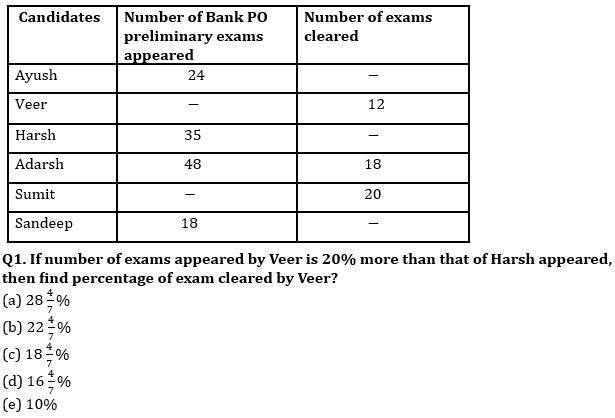Q2. Total number of exams in which Sumit appeared is 10% more than that of Veer appeared. If Sumit appeared in four less exams than Adarsh, then find number of exams, which Veer did not clear?
(a) 24
(b) 32
(c) 36
(d) 44
(e) 28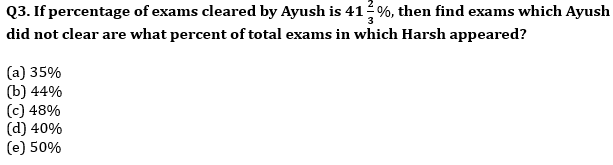Q4. If number of exams in which Sumit appeared is equal to 12 more than average number of exams in which Ayush & Adarsh appeared, then find ratio of exams which Sumit did not clear to exams which Adarsh did not clear?
(a) 14 : 17
(b) 14 :1 5
(c) 13 : 15
(d) 3 : 5
(e) 4 : 5

Q5. Total number of exams in which Sumit appeared is 37.5% more than that of Ayush appeared, while total number of exams in which Veer appeared is 20% more than that of Harsh appeared. If Sandeep cleared 50% of appeared exams, then find exams cleared by Sandeep is what percent of total exams in which all six appeared?
(a) 3.5%
(b) 4.5%
(c) 2.5%
(d) 1.5%
(e) 5%

Directions (6-10): The following table shows the total no. students and percentage of students present on a particular day out of them for two schools A and B in different classes.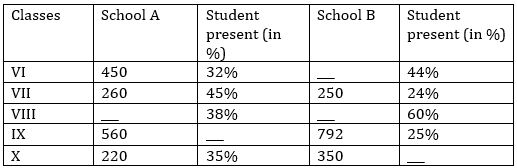Q6. If total number of students in class VIII in both the school together are 1625, while total students present in both the classes together are 766, then find difference between number of students in class VIII in both the schools?
(a)250
(b) 275
(c) 225
(d) 300
(e) None of these

Q7. The number of students present in class VI in school A are approximately what percent of number of students present in class IX in school B?
(a)65%
(b) 70%
(c) 75%
(d) 73%
(e) 80%

Q8. If number of students present in class VI in school B are 2 less than double of the number of students presents in same class in school A, then find the total number of students in class VI in school B.
(a)625
(b) 650
(c) 600
(d) 700
(e) None of these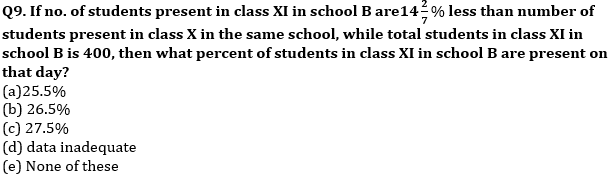Q10. Find the total number of students present in class VII in both the schools together?
(a) 157
(b) 187
(c) 167
(d) 177
(e) None of these

Directions (11-15): Study the line chart given below and answer the following questions.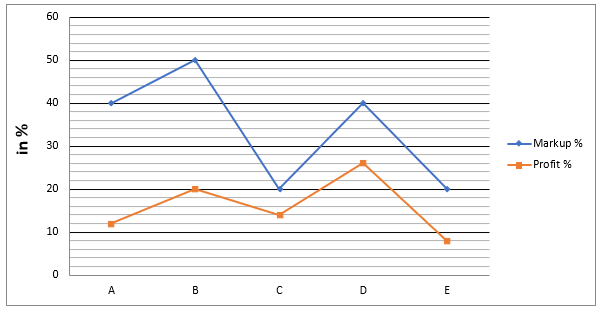Line chart shows the markup percentage with respect to cost price and profit percentage of five articles.

Q11. If discount allowed on article-C & E is Rs.48 & Rs.120 respectively, then find ratio of selling price of article-C to selling price of article-E.
(a) 38 : 45
(b) 21 : 22
(c) 5 : 12
(d) 27 : 35
(e) 2 : 3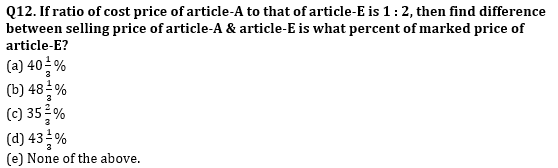Q13. If discount allowed on article-B is halved, then find the change in profit percentage.
(a) 10%
(b) 20%
(c) 15%
(d) 25%
(e) 5%

Q14. If marked price of article-C is 60% more than that of article-B and difference between discount allowed on article-B & article-C is Rs.72, then find average of cost price of article-C & B.
(a) Rs.600
(b) Rs.300
(c) Rs.500
(d) Rs.700
(e) Rs.400

Q15. If cost price of article-D is 25% of marked price of article-E, then find selling price of article-D is what percent of selling price of article-E?
(a) 25%
(b) 60%
(c) 15%
(d) 50%
(e) 35%

Solutions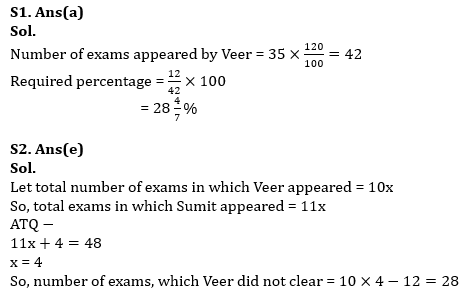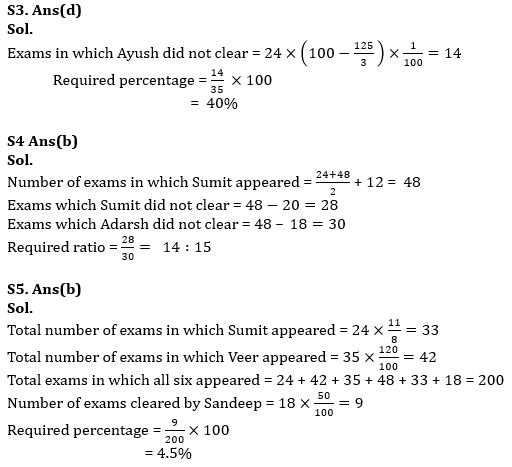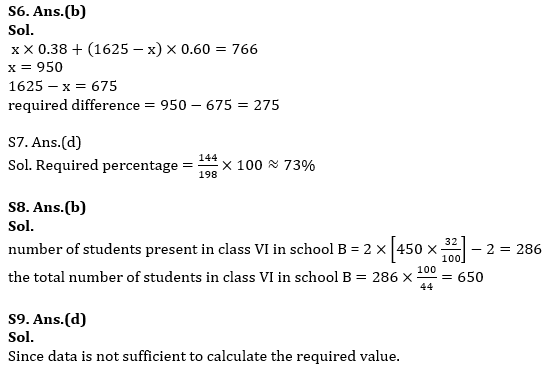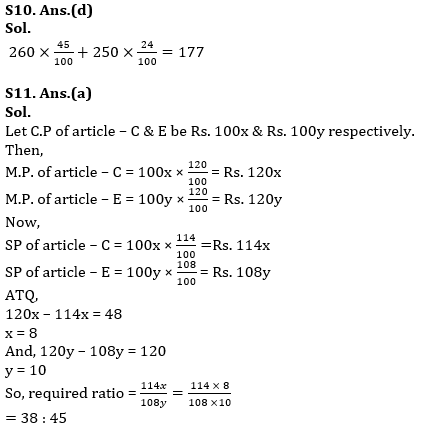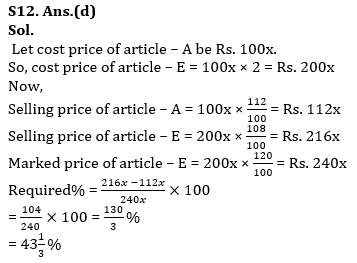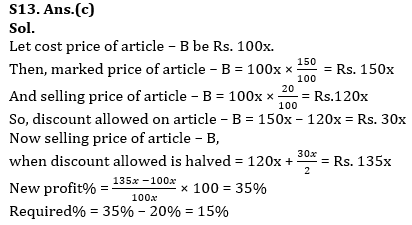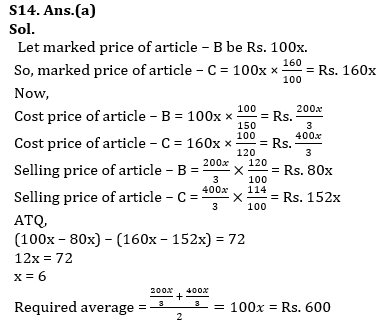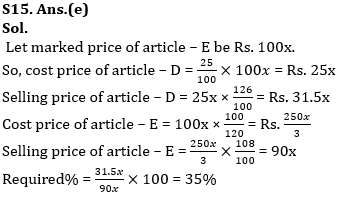## FAQs

### How many sections are there in the RBI Grade B Phase 1 Exam?

There are 4 sections in the RBI Grade B Phase 1 Exam i.e. English Language, General Awareness, Quantitative Aptitude & Reasoning.

#### Congratulations!Union Budget 2023-24: Free PDF